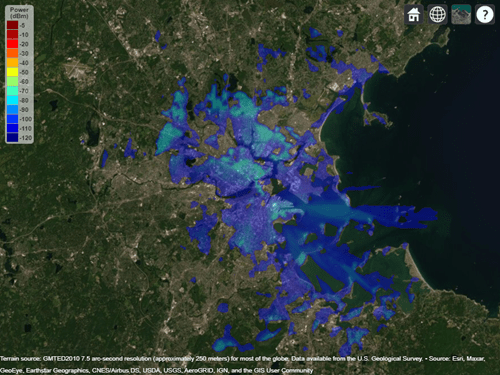# interp

Interpolate RF propagation data

Since R2020a

## Syntax

``interpvalue = interp(pd,lat,lon)``
``interpvalue = interp(pd,Name,Value)``

## Description

````interpvalue = interp(pd,lat,lon)` returns interpolated values from the propagation data for each query point specified in latitude and longitude vectors. The interpolation is performed using a scattered data interpolation method. Values corresponding to query points outside the data region are assigned a `NaN`.```

example

````interpvalue = interp(pd,Name,Value)` returns interpolated values with additional options specified by name-value pair arguments.```

## Examples

collapse all

Define names and locations of sites around Boston.

```names = ["Fenway Park","Faneuil Hall","Bunker Hill Monument"]; lats = [42.3467,42.3598,42.3763]; lons = [-71.0972,-71.0545,-71.0611];```

Create array of transmitter sites.

```txs = txsite("Name", names,... "Latitude",lats,... "Longitude",lons, ... "TransmitterFrequency",2.5e9);```

Compute received power data for each transmitter site.

```maxr = 20000; pd1 = coverage(txs(1),"MaxRange",maxr); pd2 = coverage(txs(2),"MaxRange",maxr); pd3 = coverage(txs(3),"MaxRange",maxr);```

Compute rectangle containing locations of all data.

```locs = [location(pd1); location(pd2); location(pd3)]; [minlatlon, maxlatlon] = bounds(locs);```

Create grid of locations over rectangle.

```gridlength = 300; latv = linspace(minlatlon(1),maxlatlon(1),gridlength); lonv = linspace(minlatlon(2),maxlatlon(2),gridlength); [lons,lats] = meshgrid(lonv,latv); lats = lats(:); lons = lons(:);```

Get data for each transmitter at grid locations using interpolation.

```v1 = interp(pd1,lats,lons); v2 = interp(pd2,lats,lons); v3 = interp(pd3,lats,lons);```

Create propagation data containing minimum received power values.

```minReceivedPower = min([v1 v2 v3],[],2,"includenan"); pd = propagationData(lats,lons,"MinReceivedPower",minReceivedPower);```

Plot minimum received power, which shows the weakest signal received from any transmitter site. The area shown may correspond to the service area of triangulation using the three transmitter sites.

```sensitivity = -110; contour(pd,"Levels",sensitivity:-5,"Type","power")```## Input Arguments

collapse all

Propagation data, specified as a `propagationData` object.

Latitude coordinate values, specified as a vector in decimal degrees with reference to Earth's ellipsoid. model WGS-84. The latitude coordinates must be in the range `[-90 90]`.

Longitude coordinate values, specified as a vector in decimal degrees with reference to Earth's ellipsoid. model WGS-84.

### Name-Value Arguments

Specify optional pairs of arguments as `Name1=Value1,...,NameN=ValueN`, where `Name` is the argument name and `Value` is the corresponding value. Name-value arguments must appear after other arguments, but the order of the pairs does not matter.

Before R2021a, use commas to separate each name and value, and enclose `Name` in quotes.

Example: `'Method','linear'`

Data variable to interpolate, specified as the comma-separated pair consisting of `'DataVariableName'` and a character vector or string scalar corresponding to a variable name in the data table used to create the `propagationData` container object. The default value is the `DataVariableName` property in the `propagationData`.

Data Types: `char` | `string`

Method used to interpolate data, specified as the comma separated-pair consisting `'Method'` and one of the following:

• `'natural'` - Natural neighbor interpolation

• `'linear'` - Linear interpolation

• `'nearest'` - Nearest neighbor interpolation

Data Types: `char` | `string`

## Output Arguments

collapse all

Interpolated values from the propagation data for each query point specified in latitude and longitude vectors, returned as a numeric vector.

## Version History

Introduced in R2020a